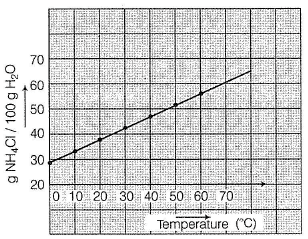# A solution of NH4CI is prepared by dissolving 95 g of NH4CI in 200 g of H2O at 60° C. What is mass per cent when the solution is cooled to 20° C based on thisa)23%b)36%c)42%d)72%Correct answer is option 'B'. Can you explain this answer? Related Test: Test: Different Methods Of Expressing Concentration Of Solutions

## Class 12 QuestionMayank Mar 27, 2019
(b) 200 g H2O at 60°C has 95 g NH4Cl
Based on solubility graph, at 20°C,
100 g of H2O can dissolve = 36 g NH4CI
hence, 200 g of H20 can dissolve = 72g  NH4CI
Thus, NH4CI separated (crystallised) = 95 - 72 = 23 g
Mass % = 36%